﻿ 一类环上的三自由度耦合van der Pol方程的同伦分析方法

# 一类环上的三自由度耦合van der Pol方程的同伦分析方法The Homotopy Analysis Method for a Class of Three-Degree-of-Freedom Coupled van der Pol Oscillators

Abstract: In this paper, the approximate expression of periodic solution for a class of 3-DOF coupled van der Pol oscillator ring system is studied by homotopy analysis method. We first give the general equation of the system, and then divide the general equation into four categories: First, all oscillators move synchronously; second, two oscillators move synchronously, while the third vibrator moves in an independent manner (except that it oscillates in the same period as the second); third, the phase difference between adjacent oscillators on the ring is one third-period motion. Fourth, the two oscillators are 1/2 cycle apart, and the third vibrates at twice their frequency. Four different types of van der Pol oscillators are used to illustrate the validity and wide application of the homotopy analysis method.

1. 引言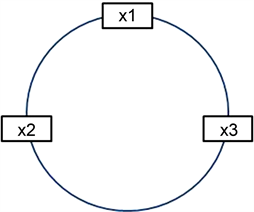Figure 1. The ring of three-degree-of-freedom van der Pol oscillators with coupled

2. 同伦分析方法简述

$M\stackrel{¨}{q}+G\stackrel{˙}{q}+Kq=F\left(q\right),$ (2-1)

$N\left[u\left(r,t\right)\right]=M\frac{{\partial }^{2}u\left(r,t\right)}{\partial {t}^{2}}+G\frac{\partial u\left(r,t\right)}{\partial t}+Ku\left(r,t\right)-F\left[u\left(r,t\right)\right],$ (2-2)

$u\left(r,t\right)={\left({x}_{1}\left(t\right),\cdots ,{x}_{n}\left(t\right)\right)}^{\text{T}},$ (2-3)

$\frac{\partial u\left(r,t\right)}{\partial t}={\left(\frac{\text{d}{x}_{1}}{\text{d}t},\cdots ,\frac{\text{d}{x}_{n}}{\text{d}t}\right)}^{\text{T}},$ (2-4)

$\frac{{\partial }^{2}u\left(r,t\right)}{\partial {t}^{2}}={\left(\frac{{\text{d}}^{2}{x}_{1}}{\text{d}{t}^{2}},\cdots ,\frac{{\text{d}}^{2}{x}_{n}}{\text{d}{t}^{2}}\right)}^{\text{T}},$ (2-5)

$\left(1-p\right)\left\{L\left[\Phi \left(r,t,p\right)-{u}_{0}\left(r,t\right)\right]\right\}=phH\left(t\right)N\left[\Phi \left(r,t,p\right)\right],$ (2-6)

${u}_{m}\left(r,t\right)=\frac{1}{m!}{\frac{{\partial }^{m}\Phi \left(r,t,p\right)}{\partial {p}^{m}}|}_{p=0}$ (2-7)

$\Phi \left(r,t,p\right)={u}_{0}\left(r,t\right)+{\sum }_{m=1}^{+\infty }{u}_{m}\left(r,t\right){p}^{m}.$ (2-8)

$u\left(r,t\right)={u}_{0}\left(r,t\right)+{\sum }_{m=1}^{+\infty }{u}_{m}\left(r,t\right)\text{ }\text{ }.$ (2-9)

${u}_{m}=\left\{{u}_{0}\left(r,t\right),{u}_{1}\left(r,t\right),\cdots ,{u}_{m}\left(r,t\right)\right\}.$ (2-10)

$L\left[{u}_{m}\left(r,t\right)-{\chi }_{m}{u}_{m-1}\left(r,t\right)\right]=hH\left(t\right){R}_{m}\left({u}_{m-1},r,t\right),$ (2-11)

${\chi }_{m}=\left\{\begin{array}{l}0,\text{\hspace{0.17em}}\text{\hspace{0.17em}}\text{\hspace{0.17em}}\text{ }m\le 1\\ 1,\text{\hspace{0.17em}}\text{\hspace{0.17em}}\text{\hspace{0.17em}}\text{\hspace{0.17em}}m>1\end{array}$ (2-12)

${R}_{m}\left({u}_{m-1},r,t\right)=\frac{1}{\left(m-1\right)!}{\frac{{\partial }^{m-1}N\left(\Phi \left(r,t,p\right)\right)}{\partial {p}^{m-1}}|}_{p=0}.$ (2-13)

3. 同伦分析方法的应用

$\begin{array}{l}{\mu }_{1}{\stackrel{¨}{x}}_{1}+{\epsilon }_{1}\left({x}_{1}^{2}-\lambda \right){\stackrel{˙}{x}}_{1}+{\eta }_{1}{x}_{1}={f}_{1}\left({x}_{1},{x}_{2},{x}_{3}\right)\\ {\mu }_{2}{\stackrel{¨}{x}}_{2}+{\epsilon }_{2}\left({x}_{2}^{2}-\lambda \right){\stackrel{˙}{x}}_{2}+{\eta }_{2}{x}_{2}={f}_{2}\left({x}_{1},{x}_{2},{x}_{3}\right)\\ {\mu }_{3}{\stackrel{¨}{x}}_{3}+{\epsilon }_{3}\left({x}_{3}^{2}-\lambda \right){\stackrel{˙}{x}}_{3}+{\eta }_{3}{x}_{3}={f}_{3}\left({x}_{1},{x}_{2},{x}_{3}\right)\end{array}$ (3-1)

$\begin{array}{l}{\mu }_{1}{\stackrel{¨}{x}}_{1}+{\epsilon }_{1}\left({x}_{1}^{2}-\lambda \right){\stackrel{˙}{x}}_{1}+{\eta }_{1}{x}_{1}={f}_{1}\left({x}_{1},{x}_{2},{x}_{3}\right)\\ {\mu }_{2}{\stackrel{¨}{x}}_{2}+{\epsilon }_{2}\left({x}_{2}^{2}-\lambda \right){\stackrel{˙}{x}}_{2}+{\eta }_{2}{x}_{2}={f}_{2}\left({x}_{1},{x}_{2},{x}_{3}\right)\\ {\mu }_{3}{\stackrel{¨}{x}}_{3}+{\epsilon }_{3}\left({x}_{3}^{2}-\lambda \right){\stackrel{˙}{x}}_{3}+{\eta }_{3}{x}_{3}={f}_{3}\left({x}_{1},{x}_{2},{x}_{3}\right)\end{array}$ (3-2)

$\begin{array}{l}{\mu }_{1}{\omega }^{2}{\stackrel{¨}{u}}_{1}+{\epsilon }_{1}\omega \left({u}_{1}^{2}-\lambda \right){\stackrel{˙}{u}}_{1}+{\eta }_{1}{u}_{1}={f}_{1}\left({u}_{1},{u}_{2},{u}_{3}\right)\\ {\mu }_{2}{\omega }^{2}{\stackrel{¨}{u}}_{2}+{\epsilon }_{2}\omega \left({u}_{2}^{2}-\lambda \right){\stackrel{˙}{u}}_{2}+{\eta }_{2}{u}_{2}={f}_{2}\left({u}_{1},{u}_{2},{u}_{3}\right)\\ {\mu }_{3}{\omega }^{2}{\stackrel{¨}{u}}_{3}+{\epsilon }_{3}\omega \left({u}_{3}^{2}-\lambda \right){\stackrel{˙}{u}}_{3}+{\eta }_{3}{u}_{3}={f}_{3}\left({u}_{1},{u}_{2},{u}_{3}\right)\end{array}$ (3-3)

${u}_{1}\left(0\right)=a,{{u}^{\prime }}_{1}\left(0\right)=b,{u}_{2}\left(0\right)=c,{{u}^{\prime }}_{2}\left(0\right)=d,{u}_{3}\left(0\right)=e,{{u}^{\prime }}_{3}\left(0\right)=0$ (3-4)

$\begin{array}{l}{u}_{1}\left(\tau \right)={\sum }_{k=0}^{+\infty }\left({a}_{1,k}\mathrm{cos}k\tau +{b}_{1,k}\mathrm{sin}k\tau \right)\\ {u}_{2}\left(\tau \right)={\sum }_{k=0}^{+\infty }\left({a}_{2,k}\mathrm{cos}k\tau +{b}_{2,k}\mathrm{sin}k\tau \right)\\ {u}_{3}\left(\tau \right)={\sum }_{k=0}^{+\infty }\left({a}_{3,k}\mathrm{cos}k\tau +{b}_{3,k}\mathrm{sin}k\tau \right)\end{array}$ (3-5)

$\begin{array}{l}{u}_{1,0}\left(\tau \right)={a}_{0}\mathrm{cos}\tau +{b}_{0}\mathrm{sin}\tau ,\\ {u}_{2,0}\left(\tau \right)={c}_{0}\mathrm{cos}\tau +{d}_{0}\mathrm{sin}\tau ,\\ {u}_{3,0}\left(\tau \right)={e}_{0}\mathrm{cos}\tau .\end{array}$ (3-6)

$L\left(\begin{array}{c}{\Phi }_{1}\left(\tau ,p\right)\\ {\Phi }_{2}\left(\tau ,p\right)\\ {\Phi }_{3}\left(\tau ,p\right)\end{array}\right)={\omega }_{0}^{2}\left(\begin{array}{c}\frac{{\partial }^{2}{\Phi }_{1}\left(\tau ,p\right)}{\partial {\tau }^{2}}+{\Phi }_{1}\left(\tau ,p\right)\\ \frac{{\partial }^{2}{\Phi }_{2}\left(\tau ,p\right)}{\partial {\tau }^{2}}+{\Phi }_{2}\left(\tau ,p\right)\\ \frac{{\partial }^{2}{\Phi }_{3}\left(\tau ,p\right)}{\partial {\tau }^{2}}+{\Phi }_{3}\left(\tau ,p\right)\end{array}\right),$ (3-7)

$L\left(\begin{array}{c}{C}_{1}\mathrm{sin}\tau +{C}_{2}\mathrm{cos}\tau \\ {C}_{3}\mathrm{sin}\tau +{C}_{4}\mathrm{cos}\tau \\ {C}_{5}\mathrm{sin}\tau +{C}_{6}\mathrm{cos}\tau \end{array}\right)=0.$ (3-8)

$\begin{array}{l}N\left(\begin{array}{c}{\Phi }_{1}\left(\tau ,p\right)\\ {\Phi }_{2}\left(\tau ,p\right)\\ {\Phi }_{3}\left(\tau ,p\right)\end{array}\right)\\ =\left(\begin{array}{c}{\mu }_{1}{\Omega }^{2}\left(p\right)\frac{{\partial }^{2}{\Phi }_{1}\left(\tau ,p\right)}{\partial {\tau }^{2}}+{\epsilon }_{1}\Omega \left(p\right)\left[{\Phi }_{1}^{2}\left(\tau ,p\right)-\lambda \right]\frac{\partial {\Phi }_{1}\left(\tau ,p\right)}{\partial \tau }+{\eta }_{1}{\Phi }_{1}\left(\tau ,p\right)-{f}_{1}\left({\Phi }_{1}\left(\tau ,p\right),{\Phi }_{2}\left(\tau ,p\right),{\Phi }_{3}\left(\tau ,p\right)\right)\\ {\mu }_{2}{\Omega }^{2}\left(p\right)\frac{{\partial }^{2}{\Phi }_{2}\left(\tau ,p\right)}{\partial {\tau }^{2}}+{\epsilon }_{2}\Omega \left(p\right)\left[{\Phi }_{2}^{2}\left(\tau ,p\right)-\lambda \right]\frac{\partial {\Phi }_{2}\left(\tau ,p\right)}{\partial \tau }+{\eta }_{2}{\Phi }_{2}\left(\tau ,p\right)-{f}_{2}\left({\Phi }_{1}\left(\tau ,p\right),{\Phi }_{2}\left(\tau ,p\right),{\Phi }_{3}\left(\tau ,p\right)\right)\\ {\mu }_{3}{\Omega }^{2}\left(p\right)\frac{{\partial }^{2}{\Phi }_{3}\left(\tau ,p\right)}{\partial {\tau }^{2}}+{\epsilon }_{3}\Omega \left(p\right)\left[{\Phi }_{3}^{2}\left(\tau ,p\right)-\lambda \right]\frac{\partial {\Phi }_{3}\left(\tau ,p\right)}{\partial \tau }+{\eta }_{3}{\Phi }_{3}\left(\tau ,p\right)-{f}_{3}\left({\Phi }_{1}\left(\tau ,p\right),{\Phi }_{2}\left(\tau ,p\right),{\Phi }_{3}\left(\tau ,p\right)\right)\end{array}\right)\end{array}$

$\left(1-p\right)L\left(\begin{array}{c}{\Phi }_{1}\left(\tau ,p\right)-{u}_{1,0}\left(\tau \right)\\ {\Phi }_{2}\left(\tau ,p\right)-{u}_{2,0}\left(\tau \right)\\ {\Phi }_{3}\left(\tau ,p\right)-{u}_{3,0}\left(\tau \right)\end{array}\right)=phN\left(\begin{array}{c}{\Phi }_{1}\left(\tau ,p\right)\\ {\Phi }_{2}\left(\tau ,p\right)\\ {\Phi }_{3}\left(\tau ,p\right)\end{array}\right),$ (3-9)

$\begin{array}{l}{\Phi }_{1}\left(0,p\right)=a\left(p\right),\text{\hspace{0.17em}}\text{\hspace{0.17em}}{\frac{\partial {\Phi }_{1}\left(\tau ,p\right)}{\partial \tau }|}_{\tau =0}=b\left(p\right),\\ {\Phi }_{2}\left(0,p\right)=c\left(p\right),\text{\hspace{0.17em}}\text{\hspace{0.17em}}{\frac{\partial {\Phi }_{2}\left(\tau ,p\right)}{\partial \tau }|}_{\tau =0}=d\left(p\right),\\ {\Phi }_{3}\left(0,p\right)=e\left(p\right),\text{\hspace{0.17em}}\text{\hspace{0.17em}}{\frac{\partial {\Phi }_{3}\left(\tau ,p\right)}{\partial \tau }|}_{\tau =0}=0.\end{array}$ (3-10)

${\Phi }_{1}\left(\tau ,0\right)={u}_{1,0}\left(\tau \right),\text{\hspace{0.17em}}\text{\hspace{0.17em}}{\Phi }_{2}\left(\tau ,0\right)={u}_{2,0}\left(\tau \right),\text{\hspace{0.17em}}\text{\hspace{0.17em}}{\Phi }_{3}\left(\tau ,0\right)={u}_{3,0}\left(\tau \right),\text{\hspace{0.17em}}\text{\hspace{0.17em}}\Omega \left(0\right)={\omega }_{0}.$ (3-11)

${\Phi }_{1}\left(\tau ,1\right)={u}_{1}\left(\tau \right),\text{\hspace{0.17em}}\text{\hspace{0.17em}}{\Phi }_{2}\left(\tau ,1\right)={u}_{2}\left(\tau \right),\text{\hspace{0.17em}}\text{\hspace{0.17em}}{\Phi }_{3}\left(\tau ,1\right)={u}_{3}\left(\tau \right),\text{\hspace{0.17em}}\text{\hspace{0.17em}}\Omega \left(1\right)=\omega .$ (3-12)

$\begin{array}{l}{\Phi }_{1}\left(\tau ,p\right)={u}_{1,0}\left(\tau \right)+{\sum }_{m=1}^{\infty }{u}_{1,m}\left(\tau \right){p}^{m},\\ {\Phi }_{2}\left(\tau ,p\right)={u}_{2,0}\left(\tau \right)+{\sum }_{m=1}^{\infty }{u}_{2,m}\left(\tau \right){p}^{m},\\ {\Phi }_{3}\left(\tau ,p\right)={u}_{3,0}\left(\tau \right)+{\sum }_{m=1}^{\infty }{u}_{3,m}\left(\tau \right){p}^{m},\\ \Omega \left(p\right)={\omega }_{0}+{\sum }_{m=1}^{\infty }{\omega }_{m}{p}^{m}\text{}\end{array}$ (3-13)

$\begin{array}{l}{u}_{1,m}\left(\tau \right)=\frac{1}{m!}{\frac{{\partial }^{m}{\Phi }_{1}\left(\tau ,p\right)}{\partial {p}^{m}}|}_{p=0},\\ {u}_{2,m}\left(\tau \right)=\frac{1}{m!}{\frac{{\partial }^{m}{\Phi }_{2}\left(\tau ,p\right)}{\partial {p}^{m}}|}_{p=0},\\ {u}_{3,m}\left(\tau \right)=\frac{1}{m!}{\frac{{\partial }^{m}{\Phi }_{3}\left(\tau ,p\right)}{\partial {p}^{m}}|}_{p=0},\\ {\omega }_{m}=\frac{1}{m!}{\frac{{\partial }^{m}\Omega \left(p\right)}{\partial {p}^{m}}|}_{p=0}.\end{array}$ (3-14)

$\begin{array}{l}{u}_{1}\left(\tau \right)={u}_{1,0}\left(\tau \right)+{\sum }_{m=1}^{\infty }{u}_{1,m}\left(\tau \right),\\ {u}_{2}\left(\tau \right)={u}_{2,0}\left(\tau \right)+{\sum }_{m=1}^{\infty }{u}_{2,m}\left(\tau \right),\\ {u}_{3}\left(\tau \right)={u}_{3,0}\left(\tau \right)+{\sum }_{m=1}^{\infty }{u}_{3,m}\left(\tau \right),\\ \omega ={\omega }_{0}+{\sum }_{m=1}^{\infty }{\omega }_{m}.\end{array}$ (3-15)

$\begin{array}{l}{u}_{1,n}=\left\{{u}_{1,0}\left(\tau \right),{u}_{1,1}\left(\tau \right),\cdots ,{u}_{1,n}\left(\tau \right)\right\},\\ {u}_{2,n}=\left\{{u}_{2,0}\left(\tau \right),{u}_{2,1}\left(\tau \right),\cdots ,{u}_{2,n}\left(\tau \right)\right\},\\ {u}_{3,n}=\left\{{u}_{3,0}\left(\tau \right),{u}_{3,1}\left(\tau \right),\cdots ,{u}_{3,n}\left(\tau \right)\right\},\\ {\omega }_{n}=\left\{{\omega }_{0},{\omega }_{1},\cdots ,{\omega }_{n}\right\}.\end{array}$ (3-16)

$L\left(\begin{array}{c}{u}_{1,m}\left(\tau \right)-{\chi }_{m}{u}_{1,m-1}\left(\tau \right)\\ {u}_{2,m}\left(\tau \right)-{\chi }_{m}{u}_{2,m-1}\left(\tau \right)\\ {u}_{3,m}\left(\tau \right)-{\chi }_{m}{u}_{3,m-1}\left(\tau \right)\end{array}\right)=h\left(\begin{array}{c}{R}_{1,m}\left({u}_{1,m-1},{\omega }_{m-1}\right)\\ {R}_{2,m}\left({u}_{2,m-1},{\omega }_{m-1}\right)\\ {R}_{3,m}\left({u}_{3,m-1},{\omega }_{m-1}\right)\end{array}\right),$ (3-17)

$\left(\begin{array}{c}{R}_{1,m}\left({u}_{1,m-1},{\omega }_{m-1}\right)\\ {R}_{2,m}\left({u}_{2,m-1},{\omega }_{m-1}\right)\\ {R}_{3,m}\left({u}_{3,m-1},{\omega }_{m-1}\right)\end{array}\right)=\frac{1}{\left(m-1\right)!}{\frac{{\partial }^{m-1}N\left(\begin{array}{c}{\Phi }_{1}\left(\tau ,p\right)\\ {\Phi }_{2}\left(\tau ,p\right)\\ {\Phi }_{3}\left(\tau ,p\right)\end{array}\right)}{\partial {p}^{m-1}}|}_{p=0},$ (3-18)

$\begin{array}{l}{u}_{1,m}\left(0\right)={a}_{m},\text{\hspace{0.17em}}\text{\hspace{0.17em}}{{u}^{\prime }}_{1,m}\left(0\right)={b}_{m},\text{\hspace{0.17em}}\text{\hspace{0.17em}}{u}_{2,m}\left(0\right)={c}_{m},\\ {{u}^{\prime }}_{2,m}\left(0\right)={d}_{m},\text{\hspace{0.17em}}\text{\hspace{0.17em}}{u}_{3,m}\left(0\right)={e}_{m},\text{\hspace{0.17em}}\text{\hspace{0.17em}}{{u}^{\prime }}_{3,m}\left(0\right)=0,\left(m\ge 1\right)\end{array}$ (3-19)

$\begin{array}{l}\frac{1}{\pi }{\int }_{0}^{2\pi }{R}_{1,m}\left({u}_{1,m-1},{\omega }_{m-1}\right)\mathrm{cos}\tau \text{d}\tau =0,\\ \frac{1}{\pi }{\int }_{0}^{2\pi }{R}_{1,m}\left({u}_{1,m-1},{\omega }_{m-1}\right)\mathrm{sin}\tau \text{d}\tau =0,\\ \frac{1}{\pi }{\int }_{0}^{2\pi }{R}_{2,m}\left({u}_{2,m-1},{\omega }_{m-1}\right)\mathrm{cos}\tau \text{d}\tau =0,\\ \frac{1}{\pi }{\int }_{0}^{2\pi }{R}_{2,m}\left({u}_{2,m-1},{\omega }_{m-1}\right)\mathrm{sin}\tau \text{d}\tau =0,\\ \frac{1}{\pi }{\int }_{0}^{2\pi }{R}_{3,m}\left({u}_{3,m-1},{\omega }_{m-1}\right)\mathrm{cos}\tau \text{d}\tau =0,\\ \frac{1}{\pi }{\int }_{0}^{2\pi }{R}_{3,m}\left({u}_{3,m-1},{\omega }_{m-1}\right)\mathrm{sin}\tau \text{d}\tau =0,\end{array}$ (3-20)

$\begin{array}{l}{R}_{1,m}\left({u}_{1,m-1},{\omega }_{m-1}\right)={\sum }_{n=1}^{{\phi }_{1}\left(m\right)}\left[{a}_{m,n}^{1}\mathrm{cos}n\tau +{b}_{m,n}^{1}\mathrm{sin}n\tau \right]\text{ }\text{ },\\ {R}_{2,m}\left({u}_{2,m-1},{\omega }_{m-1}\right)={\sum }_{n=1}^{{\phi }_{2}\left(m\right)}\left[{a}_{m,n}^{2}\mathrm{cos}n\tau +{b}_{m,n}^{2}\mathrm{sin}n\tau \right]\text{ }\text{ },\\ {R}_{3,m}\left({u}_{3,m-1},{\omega }_{m-1}\right)={\sum }_{n=1}^{{\phi }_{2}\left(m\right)}\left[{a}_{m,n}^{3}\mathrm{cos}n\tau +{b}_{m,n}^{3}\mathrm{sin}n\tau \right]\text{ }\text{ }.\end{array}$ (3-21)

$\begin{array}{l}{a}_{m,n}^{1}=\frac{1}{\pi }{\int }_{0}^{2\pi }{R}_{1,m}\left({u}_{1,m-1},{\omega }_{m-1}\right)\mathrm{sin}\left(n\tau \right)\text{d}\tau ,\\ {b}_{m,n}^{1}=\frac{1}{\pi }{\int }_{0}^{2\pi }{R}_{1,m}\left({u}_{1,m-1},{\omega }_{m-1}\right)\mathrm{sin}\left(n\tau \right)\text{d}\tau ,\\ {a}_{m,n}^{2}=\frac{1}{\pi }{\int }_{0}^{2\pi }{R}_{2,m}\left({u}_{2,m-1},{\omega }_{m-1}\right)\mathrm{cos}\left(n\tau \right)\text{d}\tau ,\\ {b}_{m,n}^{2}=\frac{1}{\pi }{\int }_{0}^{2\pi }{R}_{2,m}\left({u}_{2,m-1},{\omega }_{m-1}\right)\mathrm{sin}\left(n\tau \right)\text{d}\tau ,\\ {a}_{m,n}^{3}=\frac{1}{\pi }{\int }_{0}^{2\pi }{R}_{3,m}\left({u}_{3,m-1},{\omega }_{m-1}\right)\mathrm{cos}\left(n\tau \right)\text{d}\tau ,\\ {b}_{m,n}^{3}=\frac{1}{\pi }{\int }_{0}^{2\pi }{R}_{3,m}\left({u}_{3,m-1},{\omega }_{m-1}\right)\mathrm{sin}\left(n\tau \right)\text{d}\tau ,\end{array}$ (3-22)

${\omega }_{-}=\omega +v.$

4. 数值模拟与讨论

4.1. 所有振子都同步运动

$\begin{array}{l}{f}_{1}\left({x}_{1},{x}_{2},{x}_{3}\right)={x}_{1}-{x}_{2}\\ {f}_{2}\left({x}_{1},{x}_{2},{x}_{3}\right)={x}_{2}-{x}_{3}\\ {f}_{3}\left({x}_{1},{x}_{2},{x}_{3}\right)={x}_{3}-{x}_{1}\end{array}$ (4-1)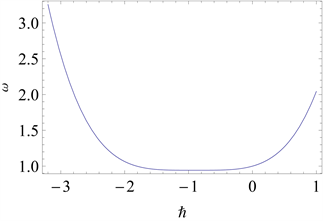Figure 2.The eighth-order approximation of versus is obtained for ${\mu }_{i}=1,{\epsilon }_{i}=1,{\eta }_{i}=1\left(i=1,2,3\right)$ and $\lambda =1$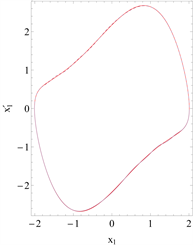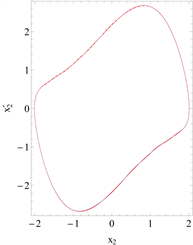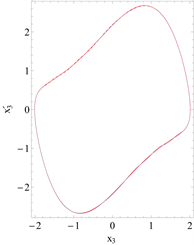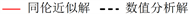Figure 3. Comparison of the phase curves of the eighth-order approximation with the numerical integration solution is given for ${\mu }_{i}=1,{\epsilon }_{i}=1,{\eta }_{i}=1,\lambda =1\left(i=1,2,3\right),{a}_{0}=2,{b}_{0}=0,{c}_{0}=2,{d}_{0}=2,{e}_{0}=2$ and ${\omega }_{0}=1$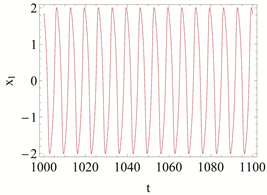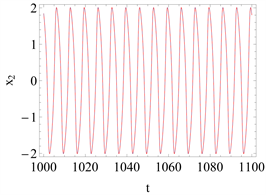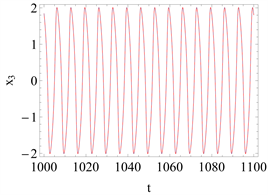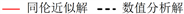Figure 4. Comparison of the time history responses of the eighth-order approximation with the numerical integration solution is given when ${\mu }_{i}=1,{\epsilon }_{i}=1,{\eta }_{i}=1,\lambda =1\left(i=1,2,3\right),{a}_{0}=2,{b}_{0}=0,{c}_{0}=2,{d}_{0}=2,{e}_{0}=2$ and ${\omega }_{0}=1$

$\begin{array}{l}{x}_{1}\left(0\right)=2.008600,\text{\hspace{0.17em}}\text{\hspace{0.17em}}{{x}^{\prime }}_{1}\left(0\right)=0,\\ {x}_{2}\left(0\right)=2.008600,\text{\hspace{0.17em}}\text{\hspace{0.17em}}{{x}^{\prime }}_{2}\left(0\right)=0,\\ {x}_{3}\left(0\right)=2.008600,\text{\hspace{0.17em}}\text{\hspace{0.17em}}{{x}^{\prime }}_{3}\left(0\right)=0,\end{array}$

$\begin{array}{c}{x}_{1}\left(\tau \right)=1.881842\mathrm{cos}\stackrel{¯}{\omega }\tau +0.720593\mathrm{sin}\stackrel{¯}{\omega }\tau +0.720593\mathrm{cos}3\stackrel{¯}{\omega }\tau -1.881842\mathrm{sin}3\stackrel{¯}{\omega }\tau \\ \text{\hspace{0.17em}}\text{\hspace{0.17em}}+0.158456\mathrm{cos}5\stackrel{¯}{\omega }\tau -0.530028\mathrm{sin}5\stackrel{¯}{\omega }\tau -0.176676\mathrm{cos}7\stackrel{¯}{\omega }\tau -0.475368\mathrm{sin}7\stackrel{¯}{\omega }\tau \\ \text{\hspace{0.17em}}\text{\hspace{0.17em}}-0.020655\mathrm{cos}9\stackrel{¯}{\omega }\tau -0.218080\mathrm{sin}9\stackrel{¯}{\omega }\tau -0.043616\mathrm{cos}11\stackrel{¯}{\omega }\tau +0.103273\mathrm{sin}11\stackrel{¯}{\omega }\tau \\ \text{\hspace{0.17em}}\text{\hspace{0.17em}}-0.010428\mathrm{cos}13\stackrel{¯}{\omega }\tau -0.000519\mathrm{sin}13\stackrel{¯}{\omega }\tau -0.000074\mathrm{cos}15\stackrel{¯}{\omega }\tau +0.072994\mathrm{sin}15\stackrel{¯}{\omega }\tau ,\end{array}$

$\begin{array}{c}{x}_{2}\left(\tau \right)=1.881842\mathrm{cos}\stackrel{¯}{\omega }\tau +0.720593\mathrm{sin}\stackrel{¯}{\omega }\tau +0.720593\mathrm{cos}3\stackrel{¯}{\omega }\tau -1.881842\mathrm{sin}3\stackrel{¯}{\omega }\tau \\ \text{\hspace{0.17em}}\text{\hspace{0.17em}}+0.158456\mathrm{cos}5\stackrel{¯}{\omega }\tau -0.530028\mathrm{sin}5\stackrel{¯}{\omega }\tau -0.176676\mathrm{cos}7\stackrel{¯}{\omega }\tau -0.475368\mathrm{sin}7\stackrel{¯}{\omega }\tau \\ \text{\hspace{0.17em}}\text{\hspace{0.17em}}-0.020655\mathrm{cos}9\stackrel{¯}{\omega }\tau -0.218080\mathrm{sin}9\stackrel{¯}{\omega }\tau -0.043616\mathrm{cos}11\stackrel{¯}{\omega }\tau +0.103273\mathrm{sin}11\stackrel{¯}{\omega }\tau \\ \text{\hspace{0.17em}}\text{\hspace{0.17em}}-0.010428\mathrm{cos}13\stackrel{¯}{\omega }\tau -0.000519\mathrm{sin}13\stackrel{¯}{\omega }\tau -0.000074\mathrm{cos}15\stackrel{¯}{\omega }\tau +0.072994\mathrm{sin}15\stackrel{¯}{\omega }\tau ,\end{array}$

$\begin{array}{c}{x}_{3}\left(\tau \right)=1.881842\mathrm{cos}\stackrel{¯}{\omega }\tau +0.720593\mathrm{sin}\stackrel{¯}{\omega }\tau +0.720593\mathrm{cos}3\stackrel{¯}{\omega }\tau -1.881842\mathrm{sin}3\stackrel{¯}{\omega }\tau \\ \text{\hspace{0.17em}}\text{\hspace{0.17em}}+0.158456\mathrm{cos}5\stackrel{¯}{\omega }\tau -0.530028\mathrm{sin}5\stackrel{¯}{\omega }\tau -0.176676\mathrm{cos}7\stackrel{¯}{\omega }\tau -0.475368\mathrm{sin}7\stackrel{¯}{\omega }\tau \\ \text{\hspace{0.17em}}\text{\hspace{0.17em}}-0.020655\mathrm{cos}9\stackrel{¯}{\omega }\tau -0.218080\mathrm{sin}9\stackrel{¯}{\omega }\tau -0.043616\mathrm{cos}11\stackrel{¯}{\omega }\tau +0.103273\mathrm{sin}11\stackrel{¯}{\omega }\tau \\ \text{\hspace{0.17em}}\text{\hspace{0.17em}}-0.010428\mathrm{cos}13\stackrel{¯}{\omega }\tau -0.000519\mathrm{sin}13\stackrel{¯}{\omega }\tau -0.000074\mathrm{cos}15\stackrel{¯}{\omega }\tau +0.072994\mathrm{sin}15\stackrel{¯}{\omega }\tau ,\end{array}$

$\omega =0.942953.$

4.2. 两个振子同步运动，而第三个振子以一无关的方式运动(除它与第二个振子有相同周期地振动外)

$\begin{array}{l}{f}_{1}\left({x}_{1},{x}_{2},{x}_{3}\right)={x}_{1}-{x}_{2}\\ {f}_{2}\left({x}_{1},{x}_{2},{x}_{3}\right)={x}_{2}-{x}_{1}\\ {f}_{3}\left({x}_{1},{x}_{2},{x}_{3}\right)={x}_{1}-{x}_{2}-10{x}_{3}\end{array}$ (4-2)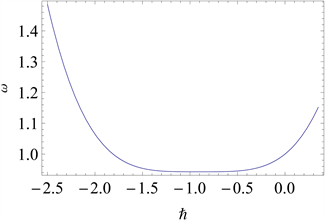Figure 5. The first-order approximation of ω versus h is obtained for ${\mu }_{i}=1,{\epsilon }_{i}=1,{\eta }_{i}=1\left(i=1,2,3\right)$ and $\lambda =1$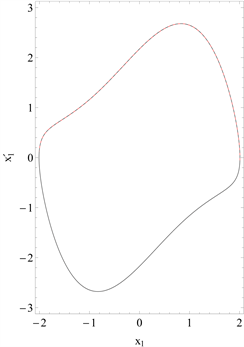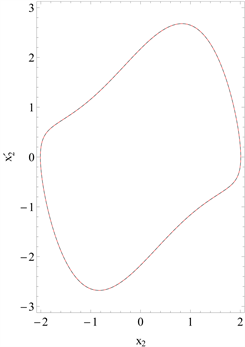Figure 6. Comparison of the phase curves of the first-order approximation with the numerical integration solution is given for ${\mu }_{i}=1,{\epsilon }_{i}=1,{\eta }_{i}=1,\lambda =1\left(i=1,2,3\right),{a}_{0}=2,{b}_{0}=0,{c}_{0}=2,{d}_{0}=2,{e}_{0}=2$ and ${\omega }_{0}=1$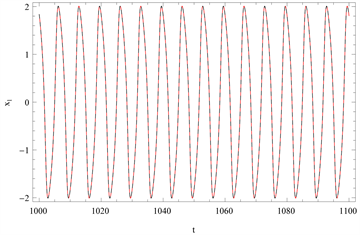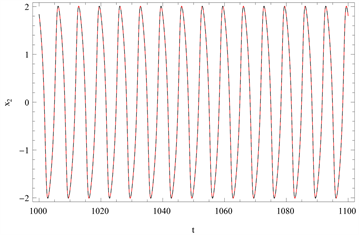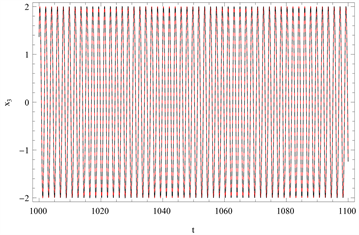Figure 7. Comparison of the time history responses of the first-order approximation with the numerical integration solution is given when ${\mu }_{i}=1,{\epsilon }_{i}=1,{\eta }_{i}=1,\lambda =1\left(i=1,2,3\right),{a}_{0}=2,{b}_{0}=0,{c}_{0}=2,{d}_{0}=2,{e}_{0}=2$ and ${\omega }_{0}=1$

$\begin{array}{c}{x}_{1}\left(\tau \right)=1.844822\mathrm{cos}\stackrel{¯}{\omega }\tau -0.118627\mathrm{sin}\stackrel{¯}{\omega }\tau -0.273745\mathrm{cos}3\stackrel{¯}{\omega }\tau -1.844822\mathrm{sin}3\stackrel{¯}{\omega }\tau \\ \text{\hspace{0.17em}}\text{\hspace{0.17em}}+0.453207\mathrm{cos}5\stackrel{¯}{\omega }\tau -0.120863\mathrm{sin}5\stackrel{¯}{\omega }\tau -1.836718\mathrm{cos}7\stackrel{¯}{\omega }\tau -0.135962\mathrm{sin}7\stackrel{¯}{\omega }\tau \\ \text{\hspace{0.17em}}\text{\hspace{0.17em}}-0.047096\mathrm{cos}9\stackrel{¯}{\omega }\tau -0.125599\mathrm{sin}9\stackrel{¯}{\omega }\tau -0.144917\mathrm{cos}11\stackrel{¯}{\omega }\tau +0.235481\mathrm{sin}11\stackrel{¯}{\omega }\tau \\ \text{\hspace{0.17em}}\text{\hspace{0.17em}}+0.500366\mathrm{cos}13\stackrel{¯}{\omega }\tau -0.003442\mathrm{sin}13\stackrel{¯}{\omega }\tau -0.021553\mathrm{cos}15\stackrel{¯}{\omega }\tau -0.035026\mathrm{sin}15\stackrel{¯}{\omega }\tau ,\end{array}$

$\begin{array}{c}{x}_{2}\left(\tau \right)=1.844822\mathrm{cos}\stackrel{¯}{\omega }\tau -0.118627\mathrm{sin}\stackrel{¯}{\omega }\tau -0.273745\mathrm{cos}3\stackrel{¯}{\omega }\tau -1.844822\mathrm{sin}3\stackrel{¯}{\omega }\tau \\ \text{\hspace{0.17em}}\text{\hspace{0.17em}}+0.453207\mathrm{cos}5\stackrel{¯}{\omega }\tau -0.120863\mathrm{sin}5\stackrel{¯}{\omega }\tau -1.836718\mathrm{cos}7\stackrel{¯}{\omega }\tau -0.135962\mathrm{sin}7\stackrel{¯}{\omega }\tau \\ \text{\hspace{0.17em}}\text{\hspace{0.17em}}-0.047096\mathrm{cos}9\stackrel{¯}{\omega }\tau -0.125599\mathrm{sin}9\stackrel{¯}{\omega }\tau -0.144917\mathrm{cos}11\stackrel{¯}{\omega }\tau +0.235481\mathrm{sin}11\stackrel{¯}{\omega }\tau \\ \text{\hspace{0.17em}}\text{\hspace{0.17em}}+0.500366\mathrm{cos}13\stackrel{¯}{\omega }\tau -0.003442\mathrm{sin}13\stackrel{¯}{\omega }\tau -0.021553\mathrm{cos}15\stackrel{¯}{\omega }\tau -0.035026\mathrm{sin}15\stackrel{¯}{\omega }\tau ,\end{array}$

$\begin{array}{c}{x}_{3}\left(\tau \right)=1.124328\mathrm{cos}\stackrel{¯}{\omega }\tau +0.573061\mathrm{sin}\stackrel{¯}{\omega }\tau +0.134443\mathrm{cos}3\stackrel{¯}{\omega }\tau -1.131251\mathrm{sin}3\stackrel{¯}{\omega }\tau \\ \text{\hspace{0.17em}}\text{\hspace{0.17em}}+0.359854\mathrm{cos}5\stackrel{¯}{\omega }\tau -0.670447\mathrm{sin}5\stackrel{¯}{\omega }\tau -0.001711\mathrm{cos}7\stackrel{¯}{\omega }\tau -0.223488\mathrm{sin}7\stackrel{¯}{\omega }\tau \\ \text{\hspace{0.17em}}\text{\hspace{0.17em}}+0.271384\mathrm{cos}9\stackrel{¯}{\omega }\tau +0.348288\mathrm{sin}9\stackrel{¯}{\omega }\tau +0.054345\mathrm{cos}11\stackrel{¯}{\omega }\tau -0.059881\mathrm{sin}11\stackrel{¯}{\omega }\tau \\ \text{\hspace{0.17em}}\text{\hspace{0.17em}}-0.171917\mathrm{cos}13\stackrel{¯}{\omega }\tau -0.066372\mathrm{sin}13\stackrel{¯}{\omega }\tau +0.167520\mathrm{cos}15\stackrel{¯}{\omega }\tau -0.089623\mathrm{sin}15\stackrel{¯}{\omega }\tau ,\end{array}$

$\omega =1.208175.$

4.3. 环上相邻的振子之间彼此都相位差1/3周期的运动

$\begin{array}{l}{f}_{1}\left({x}_{1},{x}_{2},{x}_{3}\right)=\sqrt{3}/9{\left({x}_{1}+2{x}_{2}\right)}^{3}\\ {f}_{2}\left({x}_{1},{x}_{2},{x}_{3}\right)=\sqrt{3}/9{\left({x}_{2}+2{x}_{3}\right)}^{3}\\ {f}_{3}\left({x}_{1},{x}_{2},{x}_{3}\right)=\sqrt{3}/9{\left({x}_{3}+2{x}_{1}\right)}^{3}\end{array}$ (4-3)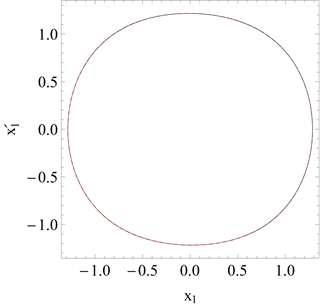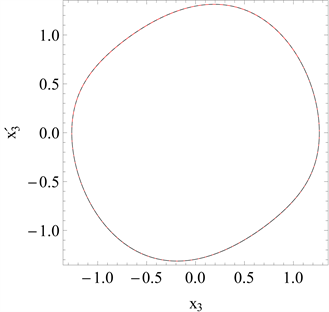Figure 8. Comparison of the phase curves of the first-order approximation with the numerical integration solution is given for ${\mu }_{i}=1,{\epsilon }_{i}=1,{\eta }_{i}=4,\lambda =1\left(i=1,2,3\right),{a}_{0}=-\sqrt{10}/5,{b}_{0}=\sqrt{30}/5,{c}_{0}=-\sqrt{10}/5,{d}_{0}=-\sqrt{30}/5,{e}_{0}=2\sqrt{10}/5$ and ${\omega }_{0}=2$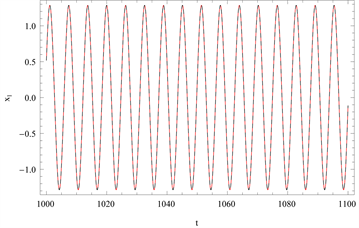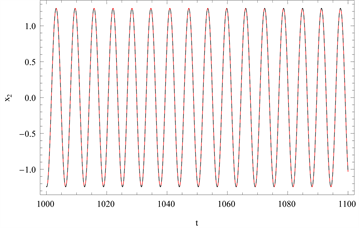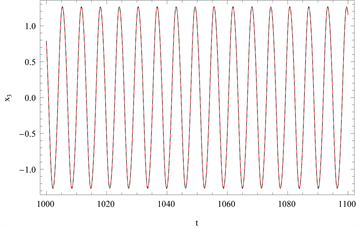Figure 9. Comparison of the time history responses of the first-order approximation with the numerical integration solution is given for ${\mu }_{i}=1,{\epsilon }_{i}=1,{\eta }_{i}=4,\lambda =1\left(i=1,2,3\right),{a}_{0}=-\sqrt{10}/5,{b}_{0}=\sqrt{30}/5,{c}_{0}=-\sqrt{10}/5,{d}_{0}=-\sqrt{30}/5,{e}_{0}=2\sqrt{10}/5$ and ${\omega }_{0}=2$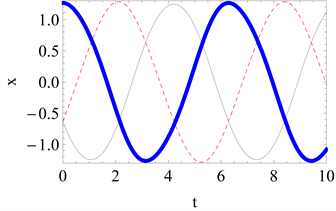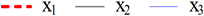Figure 10. Comparison of time history diagrams of ${x}_{1},{x}_{2}$ and ${x}_{3}$ in the same condition

${x}_{1}\left(0\right)=-0.632455,{{x}^{\prime }}_{1}\left(0\right)=1.095445,{x}_{2}\left(0\right)=-0.632455,{{x}^{\prime }}_{2}\left(0\right)=-1.095445,{x}_{3}\left(0\right)=1.264911,{{x}^{\prime }}_{3}\left(0\right)=0$

${x}_{1}\left(\tau \right)=-0.649572\mathrm{cos}\tau +1.089516\mathrm{sin}\tau +0.017116\mathrm{cos}3\tau +0.001976\mathrm{sin}3\tau ,$

${x}_{2}\left(\tau \right)=-0.615339\mathrm{cos}\tau -1.101374\mathrm{sin}\tau -0.017116\mathrm{cos}3\tau +0.001976\mathrm{sin}3\tau ,$

${x}_{3}\left(\tau \right)=1.264911\mathrm{cos}\tau +0.094868\mathrm{sin}\tau -0.031623\mathrm{sin}3\tau ,$

$\omega =2.$

4.4. 两个振子相位差1/2周期，而第三个振子2倍于它们的频率振动

$\begin{array}{l}{f}_{1}\left({x}_{1},{x}_{2},{x}_{3}\right)=\left(1-{x}_{1}^{2}\right){{x}^{\prime }}_{2}\\ {f}_{2}\left({x}_{1},{x}_{2},{x}_{3}\right)=\left(1-{x}_{2}^{2}\right){{x}^{\prime }}_{1}\\ {f}_{3}\left({x}_{1},{x}_{2},{x}_{3}\right)={x}_{1}^{3}{{x}^{\prime }}_{2}^{3}\end{array}$ (4-4)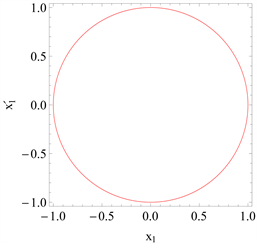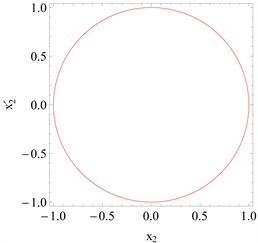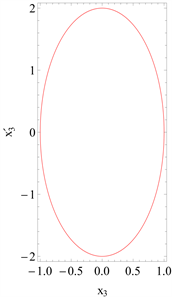Figure 11. Comparison of the phase curves of the first-order approximation with the numerical integration solution is given for ${\mu }_{i}=1,{\epsilon }_{i}=1,{\eta }_{i}=1,\lambda =1\left(i=1,2\right),{\mu }_{3}=1,{\epsilon }_{3}=1/16,{\eta }_{3}=4,{a}_{0}=1,{b}_{0}=0,{c}_{0}=-1,{d}_{0}=0,{e}_{0}=1,{\omega }_{0}=1$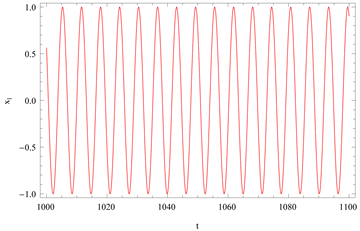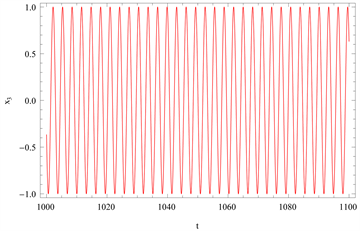Figure 12. Comparison of the time history responses of the first-order approximation with the numerical integration solution is given for ${\mu }_{i}=1,{\epsilon }_{i}=1,{\eta }_{i}=1,\lambda =1\left(i=1,2\right),{\mu }_{3}=1,{\epsilon }_{3}=1/16,{\eta }_{3}=4,{a}_{0}=1,{b}_{0}=0,{c}_{0}=-1,{d}_{0}=0,{e}_{0}=1$ and ${\omega }_{0}=1$Figure 13. Comparison of time history diagrams of ${x}_{1},{x}_{2}$ and ${x}_{3}$ in the same condition

${x}_{1}\left(0\right)=1,{{x}^{\prime }}_{1}\left(0\right)=0,{x}_{2}\left(0\right)=-1,{{x}^{\prime }}_{2}\left(0\right)=0,{x}_{3}\left(0\right)=1,{{x}^{\prime }}_{3}\left(0\right)=0$

${x}_{1}\left(\tau \right)=\mathrm{cos}\tau ,$

${x}_{2}\left(\tau \right)=-\mathrm{cos}\tau ,$

${x}_{3}\left(\tau \right)=\mathrm{cos}2\tau ,$

$\omega =1.$

5. 结论

NOTES

*通讯作者。

 Chen, H., Huang, J.L. and Sze, K.Y. (2007) Multidimensional Lindstedt-Poincare Method for Nonlinear Vibration of Axially Moving Beams. Journal of Sound and Vibration, 306, 1-11.
https://doi.org/10.1016/j.jsv.2007.05.038

 Liao, S.J. (2009) Notes on the Homotopy Analysis Method: Some Definitions and Theorems. Communication in Nonlinear Sciences and Numerical Simulation, 14, 983-997.
https://doi.org/10.1016/j.cnsns.2008.04.013

 Marinca, V. and Herisanu, N. (2010) Determination of Periodic Solutions for the Motion of a Particle on a Rotating Parabola by Means of the Optimal Homotopy Asymptotic Method. Journal of Sound and Vibration, 329, 1450-1459.
https://doi.org/10.1016/j.jsv.2009.11.005

 Liao, S.J. (2010) An Optimal Homotopy-Analysis Approach for Strongly Nonlinear Differential Equations. Communication in Nonlinear Sciences and Numerical Simulation, 15, 2003-2016.
https://doi.org/10.1016/j.cnsns.2009.09.002

 Niu, Z. and Wang, C. (2010) A One-Step Optimal Homotopy Analysis Method for Nonlinear Differential Equations. Communication in Nonlinear Sciences and Numerical Simulation, 15, 2026-2036.
https://doi.org/10.1016/j.cnsns.2009.08.014

 Chen, S.H., Chen, Y.Y. and Sze, K.Y. (2009) A Hyperbolic Perturbation Method for Determining Homoclinic Solution of Certain Strongly Nonlinear Autonomous Oscillators. Journal of Sound and Vibration, 322, 381-392.
https://doi.org/10.1016/j.jsv.2008.11.015

 廖世俊. 超越摄动: 同伦分析方法基本思想及其应用[J]. 力学进展, 2008, 38(1): 1-34.

 廖世俊. 同伦分析方法: 一种不依赖于小参数的非线性分析方法[J]. 上海力学, 1997, 18(3): 196-201.

 Liao, S.J. and Chwang, A.T. (1998) Application of Homotopy Analysis Method in Nonlinear Oscillation. ASME Journal of Application Mechanics, 65, 914-922.
https://doi.org/10.1115/1.2791935

 Rana, J. and Liao, S.J. (2019) A General Analytical Approach to Study Solute Dispersion in Non-Newtonian Fluidflow. European Journal of Mechanics B: Fluids, 77, 183-200.
https://doi.org/10.1016/j.euromechflu.2019.04.013

 Qian, Y.H., Zhang, W., Lin, B.W. and Lai, S.K. (2011) Analytical Approximate Periodic Solutions of Multi-Degree-of-Freedom Strongly Nonlinear Coupled van der Pol Oscillators by Homotopy Analysis Method. Acta Mechanica, 219, 1-14.
https://doi.org/10.1007/s00707-010-0433-3

 崔继峰. 受迫Van der Pol-Duffing振子的倍周期与拟周期之解析解研究[C]//中国力学学会动力学与控制专业委员会. 第十届动力学与控制学术会议摘要集. 中国力学学会动力学与控制专业委员会: 中国力学学会, 2016: 276.

 许天亮, 樊晓敏, 张跃进. 非线性分数阶微分方程的同伦分析解法[J]. 湘潭大学自然科学学报, 2016, 38(4): 6-9.

 郭江明. 时滞耦合van der Pol系统的双Hopf分岔与周期解的同伦方法研究[D]: [硕士学位论文]. 金华: 浙江师范大学, 2017.

 杨孟, 林炎海. 圆盘上非牛顿流体Marangoni边界层同伦分析解研究[C]//第十届全国流体力学学术会议论文摘要集. 华侨大学数学科学学院计算机科学福建省高校重点实验室, 2018: 121.

 Rompala, K., Rand, R and Howland, H. (2007) Dynamics of Three Coupled van der Pol Oscillators with Application to Circadian Rhythms. Communications in Nonlinear Science and Numerical Simulation, 12, 794-803.
https://doi.org/10.1016/j.cnsns.2005.08.002

 Tang, J.S. and Han, F. (2009) Amplitude Control of a Limit Cycle in a Coupled van der Pol System. Nonlinear Analysis: Theory, Methods & Applications, 7, 2491-2496.
https://doi.org/10.1016/j.na.2009.01.130

 钱有华, 张伟, 姚志刚. 参数激励非自治薄板系统周期解的同伦分析方法[C]//第十二届全国非线性振动暨第九届全国非线性动力学和运动稳定性学术会议论文集. 2009.

 吴俊. 两个耦合Van der Pol方程拟周期解的存在性[D]: [硕士学位论文]. 长沙: 湖南师范大学, 2016.

 石兆羽, 杨绍普, 赵志宏. 基于耦合van der Pol-Duffing系统的微弱信号检测研究[J]. 中国测试, 2018, 44(8): 107-112.

 施添添, 茅晓晨. 时滞耦合van der Pol-Duffing振子环的动力学分析[J]. 动力学与控制学报, 2019, 17(3): 264-269.

 Vinod, V., Bipin, B., Narayanan, M.D. and Mihir, S. (2015) Effect of Oscillator and Initial Condition Differences in the Dynamics of a Ring of Dissipative Coupled van der Pol Oscillators. Journal of Mechanical Science and Technology, 29, 1931-1939.
https://doi.org/10.1007/s12206-015-0103-4

 Barron, M.A. (2016) Stability of a Ring of Coupled van der Pol Oscillators with Non-Uniform Distribution of the Coupling Parameter. Journal of Applied Research and Technology, 14, 62-66.
https://doi.org/10.1016/j.jart.2016.01.002

 Fuad, R., Lu, B.L. and Surendra, S. (2007) Synchronized and Unsynchronized Chaos in a Modulated Bidirectional Ring Laser. Physical Review A: Atomic, Molecular and Optical Physics, 75, Article ID: 043811.
https://doi.org/10.1103/PhysRevA.75.043811

 Fernando, A., Ana, P.S.D. and Carla, M.A.P. (2010) Quasi-Periodic States in Coupled Rings of Cells. Communications in Nonlinear Science and Numerical Simulation, 15, 1048-1062.
https://doi.org/10.1016/j.cnsns.2009.05.035

 Faralli, S., Gambini, F., et al. (2016) Bidirectional Transmission in an Optical Network on Chip with Bus and Ring Topologies. IEEE Photonics Journal, 8, Article No. 0600407.
https://doi.org/10.1109/JPHOT.2016.2526607

 Xu, X., Yu, D.Y. and Wang, Z.H. (2019) Inter-Layer Synchronization of Periodic Solutions in Two Coupled Rings with Time Delay. Physica D: Nonlinear Phenomena, 396, 1-11.
https://doi.org/10.1016/j.physd.2019.02.010

 张锁春. 可激励系统分析的数学理论[M]. 北京: 科学出版社, 2010.

 段春梅. 一类耦合van der Pol系统的改进同伦分析方法及应用[D]: [硕士学位论文]. 金华: 浙江师范大学, 2012.

 Nohara, B.T. and Arimoto, A. (2009) Nonexistence Theorem Except the Out-of-Phase and in-Phase Solutions in the Coupled van der Pol Equation System. Ukrainian Mathematical Journal, 61, 1311-1337.
https://doi.org/10.1007/s11253-010-0278-x

 Qian, Y.H. and Chen, S.M. (2010) Accurate Approximate Analytical Solutions for Multi-Degree-of-Freedom Coupled van der Pol-Duffing Oscillators by Homotopy Analysis Method. Communications in Nonlinear Science and Numerical Simulation, 15, 3113-3130.
https://doi.org/10.1016/j.cnsns.2009.11.027

Top